Test: Areas of Similar Triangles

# Test: Areas of Similar Triangles - Class 10

Test Description

## 10 Questions MCQ Test Online MCQ Tests for Class 10 - Test: Areas of Similar Triangles

Test: Areas of Similar Triangles for Class 10 2023 is part of Online MCQ Tests for Class 10 preparation. The Test: Areas of Similar Triangles questions and answers have been prepared according to the Class 10 exam syllabus.The Test: Areas of Similar Triangles MCQs are made for Class 10 2023 Exam. Find important definitions, questions, notes, meanings, examples, exercises, MCQs and online tests for Test: Areas of Similar Triangles below.
Solutions of Test: Areas of Similar Triangles questions in English are available as part of our Online MCQ Tests for Class 10 for Class 10 & Test: Areas of Similar Triangles solutions in Hindi for Online MCQ Tests for Class 10 course. Download more important topics, notes, lectures and mock test series for Class 10 Exam by signing up for free. Attempt Test: Areas of Similar Triangles | 10 questions in 10 minutes | Mock test for Class 10 preparation | Free important questions MCQ to study Online MCQ Tests for Class 10 for Class 10 Exam | Download free PDF with solutions
 1 Crore+ students have signed up on EduRev. Have you?
Test: Areas of Similar Triangles - Question 1

### In similar triangles ABC and DEF, AG and DH are the medians. What is the value of AG/DH  given that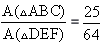​

Detailed Solution for Test: Areas of Similar Triangles - Question 1

If two triangles are similar, then the ratio of the area of both triangles is proportional to the square of the ratio of their corresponding sides. So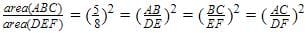Also
ΔABGand ΔDEFare also similar triangles , since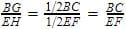, so by SAS criterion they are similar.And we know ratios of sides is ⅝ , so AG/DH=5/8

Test: Areas of Similar Triangles - Question 2

### ΔABC ~ ΔPQR . If ar (ΔABC) = 2.25 m2, ar (ΔPQR) = 6.25 m2, PQ = 0.5 m, then length of AB is:​

Test: Areas of Similar Triangles - Question 3

### Triangle ABC is similar to triangle DEF and their areas are 64 cm2 and 121 cm2 respectively. If EF = 15.4 cm, then BC = ?​

Detailed Solution for Test: Areas of Similar Triangles - Question 3

By Similar Triangle Theorem we get,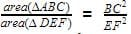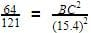By taking square roots of both sides,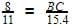BC=11.2

Test: Areas of Similar Triangles - Question 4

In ΔDEF and ΔPQR, ∠D = 30°, ∠P = 30°, ∠E = 50°, ∠Q = 50° DE = 7 cm, PQ = 13cm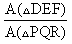= ?​

Test: Areas of Similar Triangles - Question 5

The ratio of the areas of two similar triangles is equal to the:​

Test: Areas of Similar Triangles - Question 6

Triangle ABC is similar to triangle DEF and their areas are respectively 64 cm2and 121 cm2. If EF = 15.4 cm, then BC =​

Test: Areas of Similar Triangles - Question 7

Two similar triangles ABC and DEF are such that, AB = 5cm, DE = 12cm, then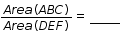Test: Areas of Similar Triangles - Question 8

Two similar triangles ABC and EDF are such that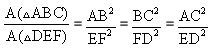Which of the following is true?​

Test: Areas of Similar Triangles - Question 9

Sides of two similar triangles are in the ratio 4: 9. What is the ratio of the area of these triangles?​

Test: Areas of Similar Triangles - Question 10

Two similar triangles ABC and DEF are such that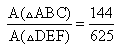.  What is the ratio of their corresponding sides?​

## Online MCQ Tests for Class 10

615 tests
Information about Test: Areas of Similar Triangles Page
In this test you can find the Exam questions for Test: Areas of Similar Triangles solved & explained in the simplest way possible. Besides giving Questions and answers for Test: Areas of Similar Triangles, EduRev gives you an ample number of Online tests for practice

615 tests